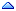Home » RDBMS Server » Performance Tuning » SQL optimisation
SQL optimisation Mon, 23 July 2012 11:38rachcha Messages: 4Registered: March 2011 Junior Member

Currently query fetch the results in 30mins.

Query:
------
select
as_at_date,
ex.h_int_ent_id,
asset_class_id,
int_entity_id,
(case when aclm_residual_maturity_days < 913 then '< 2.5 Years' else '>= 2.5 Years' end) as slotting_residual_maturity,
ex.product_id,
decode((select regulatory_product_type from d_product where product_id=ex.product_id),'PR_EQ_CIU','AIRB - Equity Simple Risk Weight',decode((select central_pd_model from d_central_pd_model where central_pd_model_id=ex.central_pd_model_id),'SRW','AIRB - Equity Simple Risk Weight','AIRB - PD/LGD Approach')) as rwa_calc_method,
sum((CASE WHEN (select aclm_product_type from d_asset_class where asset_class_id = ex.asset_class_id) in ('Project Finance','Income Producing Real Estate')
OR (decode((select regulatory_product_type from d_product where product_id=ex.product_id),'PR_EQ_CIU','AIRB - Equity Simple Risk Weight',decode((select central_pd_model from d_central_pd_model where central_pd_model_id=ex.central_pd_model_id),'SRW','AIRB - Equity Simple Risk Weight','AIRB - PD/LGD Approach')) )= 'AIRB - Equity Simple Risk Weight' THEN B2_APP_RISK_WEIGHT_RATIO
ELSE NULL
END) )IRB_RISK_WEIGHT,
sum((nvl(b2_app_rwa_post_crm_amt,0))) as b2_app_rwa_post_crm_amt,
sum(nvl(b2_app_expected_loss_amt,0)) as b2_app_expected_loss_amt,
sum(nvl(undrawn_commitment_amt,0)) as undrawn_commitment_amt,
sum(nvl(off_balance_exposure,0)) as off_balance_exposure,
sum(nvl(undrawn_commitment_amt_pst_sec,0)) as undrawn_commitment_amt_pst_sec,
sum(nvl(off_bal_led_exp_gbp_post_sec,0)) as off_bal_led_exp_gbp_post_sec,
sum(nvl(accrued_int_on_bal_amt,0)) as accrued_int_on_bal_amt,
sum(nvl(on_balance_ledger_exposure,0)) as on_balance_ledger_exposure,
sum(nvl(on_bal_led_exp_gbp_post_sec,0)) as on_bal_led_exp_gbp_post_sec,
b2_irb_effective_maturity_yrs as b2_irb_effective_maturity_yrs,
sum(nvl(cva_charge,0)) as cva_charge,
sum(nvl(coll_good_prov_amt,0)) as coll_good_prov_amt,
sum(nvl(provision_amt,0)) as provision_amt,
sum(nvl(gross_dep_utilisation_amt,0)) as gross_dep_utilisation_amt,
sum(nvl(net_dep_utilisation_amt,0)) as net_dep_utilisation_amt
, (case when (select aclm_product_type from d_asset_class where asset_class_id = ex.asset_class_id) in ('RETAIL','ON_BALANCE') AND 'N' = 'Y' THEN 0
WHEN (select aclm_product_type from d_asset_class where asset_class_id = ex.asset_class_id) in ('UNDRAWN-COMM','OFF_BALANCE') THEN 0
, (CASE WHEN (select aclm_product_type from d_asset_class where asset_class_id = ex.asset_class_id) in ('RETAIL','ON_BALANCE') AND 'N' = 'Y' THEN 0
WHEN (select aclm_product_type from d_asset_class where asset_class_id = ex.asset_class_id) in ('UNDRAWN-COMM','OFF_BALANCE') THEN sum(nvl(B2_APP_EAD_POST_CRM_AMT,0))
ELSE 0
, (CASE WHEN (select aclm_product_type from d_asset_class where asset_class_id = ex.asset_class_id) in ('RETAIL','ON_BALANCE') AND 'N' = 'Y' THEN 0
WHEN (select aclm_product_type from d_asset_class where asset_class_id = ex.asset_class_id) in ('UNDRAWN-COMM','OFF_BALANCE') THEN 0
ELSE sum(nvl(B2_APP_EXPECTED_LOSS_AMT,0))
END) EL_ON_BAL
, (CASE WHEN (select aclm_product_type from d_asset_class where asset_class_id = ex.asset_class_id) in ('RETAIL','ON_BALANCE') AND 'N' = 'Y' THEN 0
WHEN (select aclm_product_type from d_asset_class where asset_class_id = ex.asset_class_id) in ('UNDRAWN-COMM','OFF_BALANCE') THEN sum(nvl(B2_APP_EXPECTED_LOSS_AMT,0))
ELSE 0
END) EL_OFF_BAL
, (CASE WHEN (select aclm_product_type from d_asset_class where asset_class_id = ex.asset_class_id) in ('RETAIL','ON_BALANCE') AND 'N' = 'Y' THEN 0
WHEN (select aclm_product_type from d_asset_class where asset_class_id = ex.asset_class_id) in ('UNDRAWN-COMM','OFF_BALANCE') THEN 0
ELSE sum(nvl(B2_APP_RWA_POST_CRM_AMT,0))
END) RWA_ON_BAL
, (CASE WHEN (select aclm_product_type from d_asset_class where asset_class_id = ex.asset_class_id) in ('RETAIL','ON_BALANCE') AND 'N' = 'Y' THEN 0
WHEN (select aclm_product_type from d_asset_class where asset_class_id = ex.asset_class_id) in ('UNDRAWN-COMM','OFF_BALANCE') THEN sum(nvl(B2_APP_RWA_POST_CRM_AMT,0))
ELSE 0
END) RWA_OFF_BAL,
sum(nvl((select sum(decode(corep_crm_category,'GUARANTEES',nvl(B2_IRB_GTEE_AMT,0),0)) from f_exp_crm where as_at_date='29-feb-2012' and deal_id=ex.deal_id AND adjusted_flag='U' ),0)) as crm_ufcp_guar_amt,
sum(nvl((select sum(decode(corep_crm_category,'CREDIT DERIVATIVES',nvl(B2_IRB_GTEE_AMT,0),0)) from f_exp_crm where as_at_date='29-feb-2012' and deal_id=ex.deal_id AND adjusted_flag='U'),0)) as crm_ufcp_cred_deriv_amt,
sum(nvl((select sum(decode(corep_crm_category,'OTHER FUNDED CREDIT PROTECTION',nvl(B2_IRB_COLL_AMT,0),0)) from f_exp_crm where as_at_date='29-feb-2012' and deal_id=ex.deal_id AND adjusted_flag='U'),0)) as crm_fcp_other_funded_amt,
sum(nvl((select sum(decode(corep_crm_category,'REAL ESTATE',nvl(B2_IRB_COLL_AMT,0),0)) from f_exp_crm where as_at_date='29-feb-2012' and deal_id=ex.deal_id AND adjusted_flag='U'),0)) as crm_fcp_real_estate_amt,
sum(nvl((select sum(decode(COLLATERAL_TYPE,'CR',nvl(B2_IRB_COLL_AMT,0),0)) from f_exp_crm, d_crm where as_at_date='29-feb-2012' and deal_id=ex.deal_id AND adjusted_flag='U' and f_exp_crm.crm_id=d_crm.crm_id),0)) as crm_fcp_real_estate_amt,
sum(nvl((select sum(decode(corep_crm_category,'OTHER PHYSICAL COLLATERAL',nvl(B2_IRB_COLL_AMT,0),0)) from f_exp_crm where as_at_date='29-feb-2012' and deal_id=ex.deal_id AND adjusted_flag='U'),0)) as crm_fcp_oth_phy_coll_amt,
sum(nvl((select sum(decode(corep_crm_category,'RECEIVABLES',nvl(B2_IRB_GTEE_AMT,0),0)) from f_exp_crm where as_at_date='29-feb-2012' and deal_id=ex.deal_id AND adjusted_flag='U'),0)) as crm_fcp_receivables_amt,
sum(nvl((select sum(decode(corep_crm_category,'ELIGIBLE FINANCIAL COLLATERAL',nvl(B2_IRB_GTEE_AMT,0),0)) from f_exp_crm where as_at_date='29-feb-2012' and deal_id=ex.deal_id AND adjusted_flag='U'),0)) as crm_fcp_elig_fin_coll_amt,
SUM(decode(EXTERNAL_ENTITY_ID,-1,OBLIGORS_COUNT,0)) as RETAIL_OBLIGOR_COUNT,
count(distinct (decode(EXTERNAL_ENTITY_ID,-1,0,EXTERNAL_ENTITY_ID))) as CORP_OBLIGOR_COUNT,
sum(decode(EXTERNAL_ENTITY_ID,-1,'RETAIL','CORP')) as obligor_type
from rra_fact_owner.f_exp_det ex
where
exists (select 1 from d_asset_class where exposure_type_desc in ('Central Governments and Central Banks','Corporates','Equities','Institutions','Retail') and asset_class_id=ex.asset_class_id )
and not exists (select 1 from D_flags where internal_trns_flag='Y' and flags_id=ex.flags_id )
and meta_basel_data_flag='Y'
and nvl(reporting_regulator,'UK-FSA')='UK-FSA'
and approved_approach_id=4001
and date_id=387692
group by
as_at_date,
ex.h_int_ent_id,
asset_class_id,
int_entity_id,
(case when aclm_residual_maturity_days < 913 then '< 2.5 Years' else '>= 2.5 Years' end),
product_id,
central_pd_model_id,
flags_id,
asset_class_id,
b2_irb_effective_maturity_yrs

Count of tables:
------------------
select count(1) from d_product -- 65748
select count(1) from d_pd_band -- 100005
select count(1) from D_flags -- 2671028
select count(1) from d_asset_class --167802
select count(1) from f_exp_det --26883426
select count(1) from f_exp_crm --2974040

Thank you.

Re: SQL optimisation [message #561539 is a reply to message #561537] Mon, 23 July 2012 11:48Michel Cadot Messages: 66936Registered: March 2007 Location: Nanterre, France, http://... Senior MemberAccount Moderator

Michel Cadot wrote on Fri, 04 March 2011 11:36
Michel Cadot wrote on Fri, 04 March 2011 11:18
...
Make sure that lines of code do not exceed 80 characters when you format.
Indent the code, use code tags and align the columns in result.
Use the "Preview Message" button to verify.
Also always post your Oracle version, with 4 decimals.
...

For any performances question, please read http://www.orafaq.com/forum/mv/msg/84315/433888/102589/#msg_433888 and post the required information.

Regards
Michel

[Updated on: Mon, 23 July 2012 11:49]

Report message to a moderator

 Previous Topic: Sentence tuning Next Topic: Undo advice.
Goto Forum:

Current Time: Tue Apr 07 15:53:57 CDT 2020